﻿ 基于MATLAB的双声道语音信号分析及处理系统 Two-Way Sound Signals Analysis and Procession System Based on MATLAB-GUI

Open Journal of Acoustics and Vibration
Vol.05 No.02(2017), Article ID:20971,7 pages
10.12677/OJAV.2017.52005

Two-Way Sound Signals Analysis and Procession System Based on MATLAB-GUI

Xiuhui Chen, Yuxuan Zhao, Kai Qin, Jing Wang*

College of Electronic Science and Engineering, Nanjing University of Posts and Telecommunications, Nanjing Jiangsu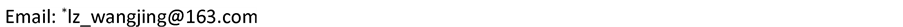Received: May 24th, 2017; accepted: Jun. 12th, 2017; published: Jun. 15th, 2017ABSTRACT

A GUI for signal procession system for single-way or two-way sounds is presented, based on MATLAB, where various operations can be carried out such as analysing sound signals, adding noise, filtering by window function method. On account of the noise at different frequencies added to the original sound signal, applicable window function can be utilized to complete filtration independently. This experiment manifests that it is convenient to understand sound signals and filter’s characteristics on this GUI, because of its simplified procedures and friendly interface, which makes this GUI perfect for teaching use.

Keywords:Two-Way, Sound Signal, FIR Filter, Window Function, MATLAB-GUI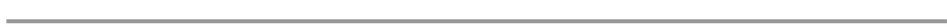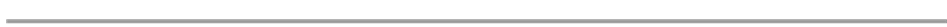1. 引言

2. 系统框图

3. 系统模块设计

3) 滤波器设计模块。利用MATLAB工具箱自带的fir1函数  和kaiserord函数  ，采用窗函数法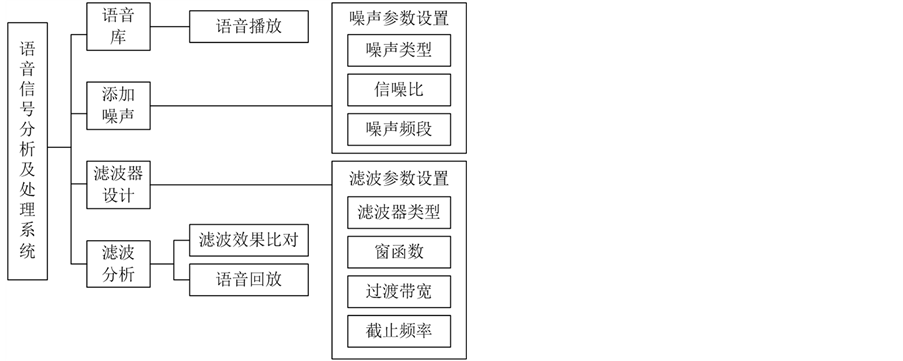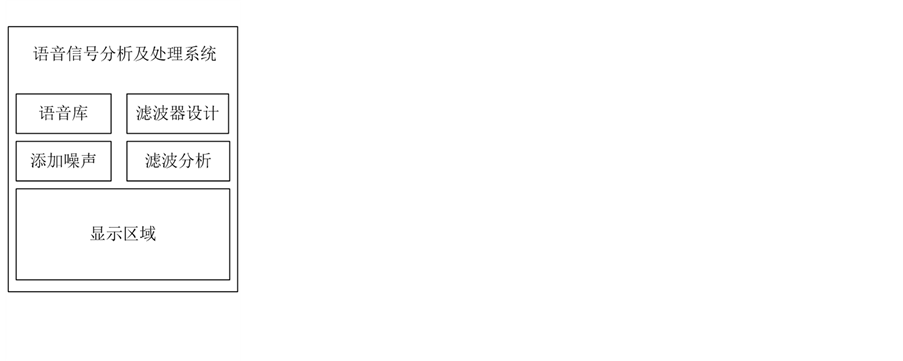(a) (b)

Figure 1. System realization block diagram; (a) Layout of main interface; (b) Modular structure

4) 滤波分析模块。调用MATLAB工具箱的fftfilt函数  完成滤波，调用plot函数画出滤波后信号的时域图和频域图，调用freqz函数  查看所设计滤波器的幅频及相位特性。

4. 图形用户界面操作

4.1. 主界面操作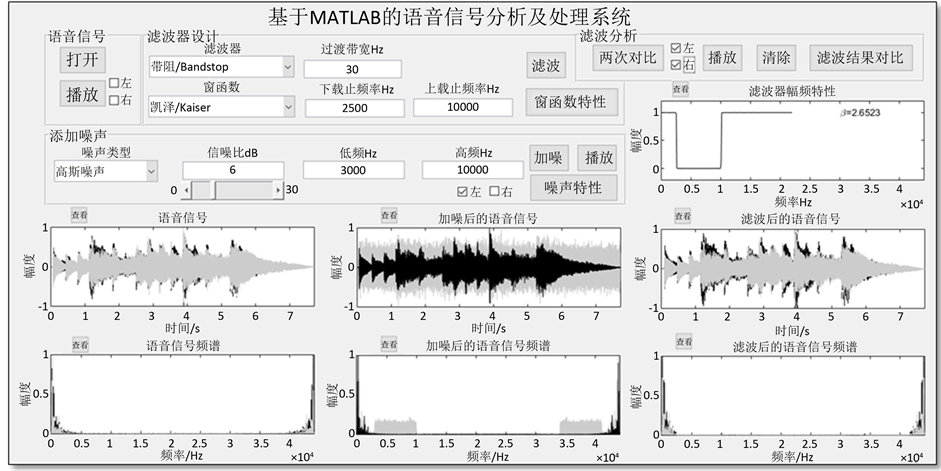Figure 2. System main interface

4.2. 子界面操作

5. 结论分析

5.1. 滤波特性

5.2. 滤波器窗函数分析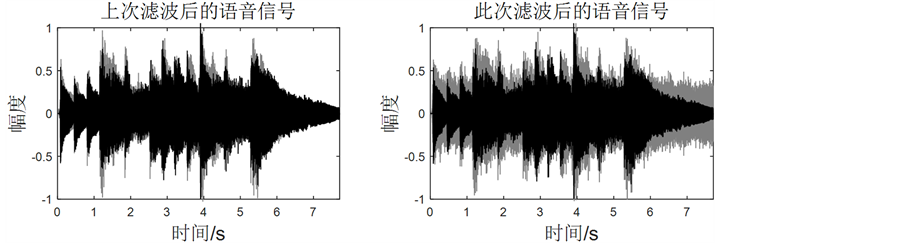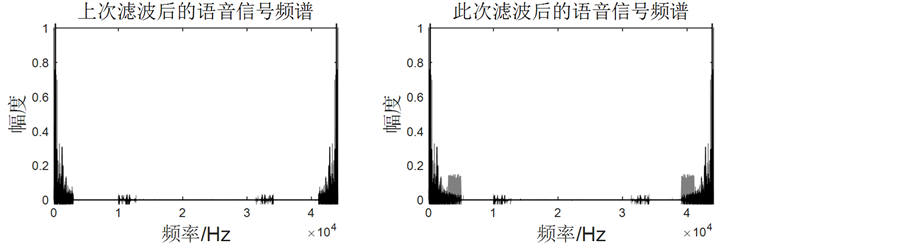Figure 3. Comparison between the result of this filtering operation and the last one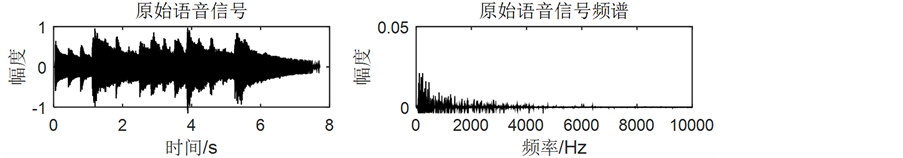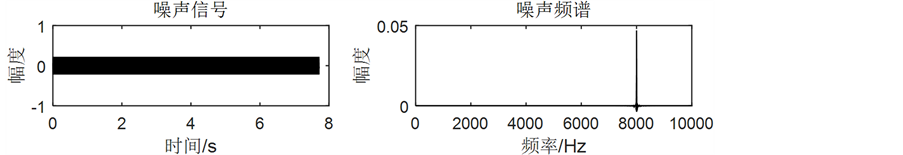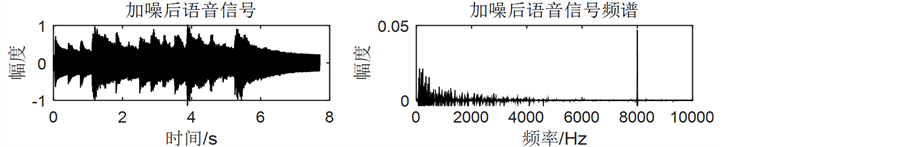Figure 4. Effect of a single-frequency noise on sounds

6. 结束语Figure 5. Amplitude-frequency characteristics of window functions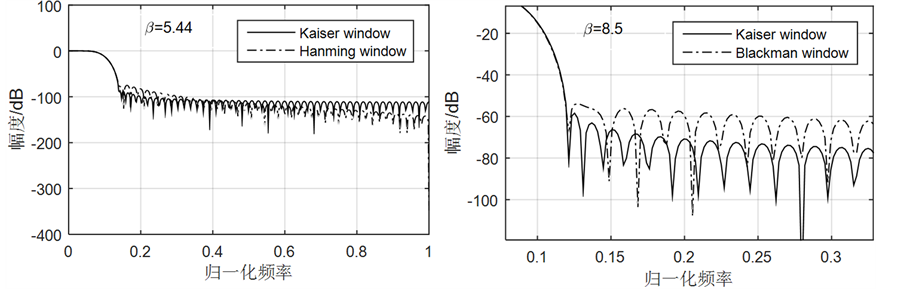Figure 6. Comparing Kaiser window’s amplitude-frequency characteristic with others

Two-Way Sound Signals Analysis and Procession System Based on MATLAB-GUI[J]. 声学与振动, 2017, 05(02): 27-33. http://dx.doi.org/10.12677/OJAV.2017.52005

1. 1. 姚天任. 数字语音处理[M]. 武汉: 华中科技大学出版社, 1992.

2. 2. 王彬, 于丹, 汪洋. MATLAB数字信号处理[M]. 北京: 机械工业出版社, 2010.

3. 3. 张德丰. MATLAB数字信号处理与应用[M]. 北京: 清华大学出版社, 2010.

4. 4. 赵颖, 刘祖深, 等. 基于MATLAB的FIR数字滤波器的方法设计[J]. 国外电子测量技术, 2012, 31(10): 35-37.

5. 5. 徐靖涛, 王金根. 基于MATLAB的语音信号分析和处理[J]. 重庆科技学院学报(自科版), 2008, 10(1): 132-136.

6. 6. 冯浩. 基于MATLABGUI的FIR数字滤波器语音信号去噪处理[J]. 菏泽学院学报, 2016, 38(5): 68-72.

7. 7. 陈然, 张霞萍. 基于MATLAB GUI的FIR数字滤波器设计与仿真[J]. 南京晓庄学院学报, 2015(6): 15-20.

8. 8. 申艳, 陈后金, 薛健, 郝晓莉, 周航. 基于Matlab加噪语音的FIR滤波器设计[J]. 电气电子教育学报, 2011, 33(2): 41-44.

9. 9. 窦海鹏. 数字滤波语音信号除噪设计[J]. 长春工业大学学报(自然科学版), 2013, 34(6): 663-667.

10. 10. 张学敏, 吕晓丽, 钟菲. 基于MATLAB图形用户界面的语音去噪分析实现[J]. 长春工程学院学报(自然科学版), 2015, 16(4): 97-99.

11. 11. 周玲. 基于MATLAB的语音信号数字滤波处理[J]. 安庆师范学院学报, 2011, 17(3): 46-49.

12. 12. 冯彦君, 靳鸿, 章晓眉. 应用MATLAB分析语音信号[J]. 光电技术应用, 2011, 26(3): 57-60.

13. 13. 张康, 刘雅. 基于MATLAB的音频文件数字滤波处理[J]. 计算机与现代化, 2007(12): 98-100.

14. 14. 余成波, 陶红艳, 杨菁, 等. 数字信号处理及MATLAB实现[M]. 第二版. 北京: 清华大学出版社, 2007.

15. 15. 从玉良. 数字信号处理原理及其MATLAB实现[M]. 北京: 电子工业出版社, 2015.

16. 16. 杨述斌, 李永全. 数字信号处理实践教程[M]. 武汉: 华中科技大学出版社, 2007.